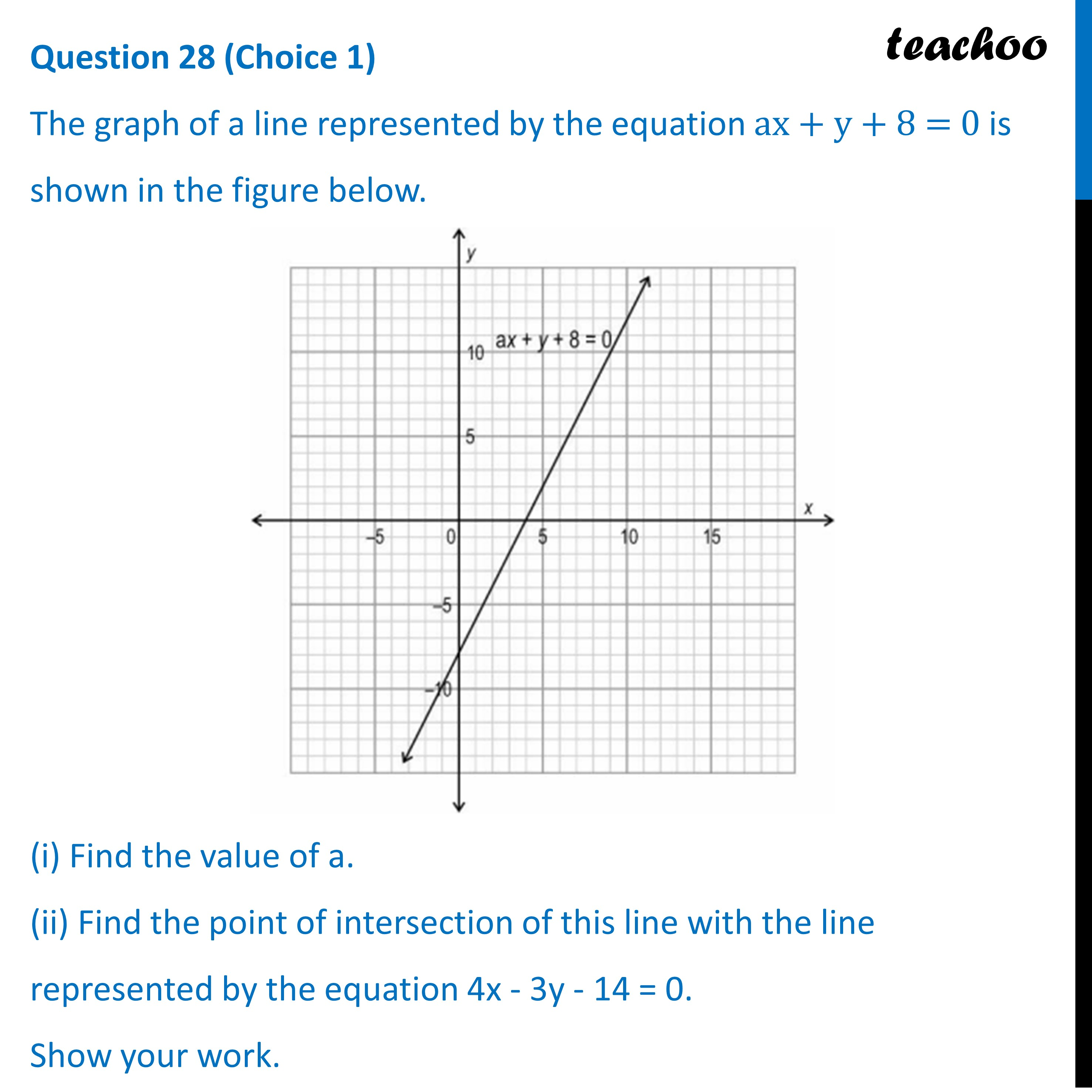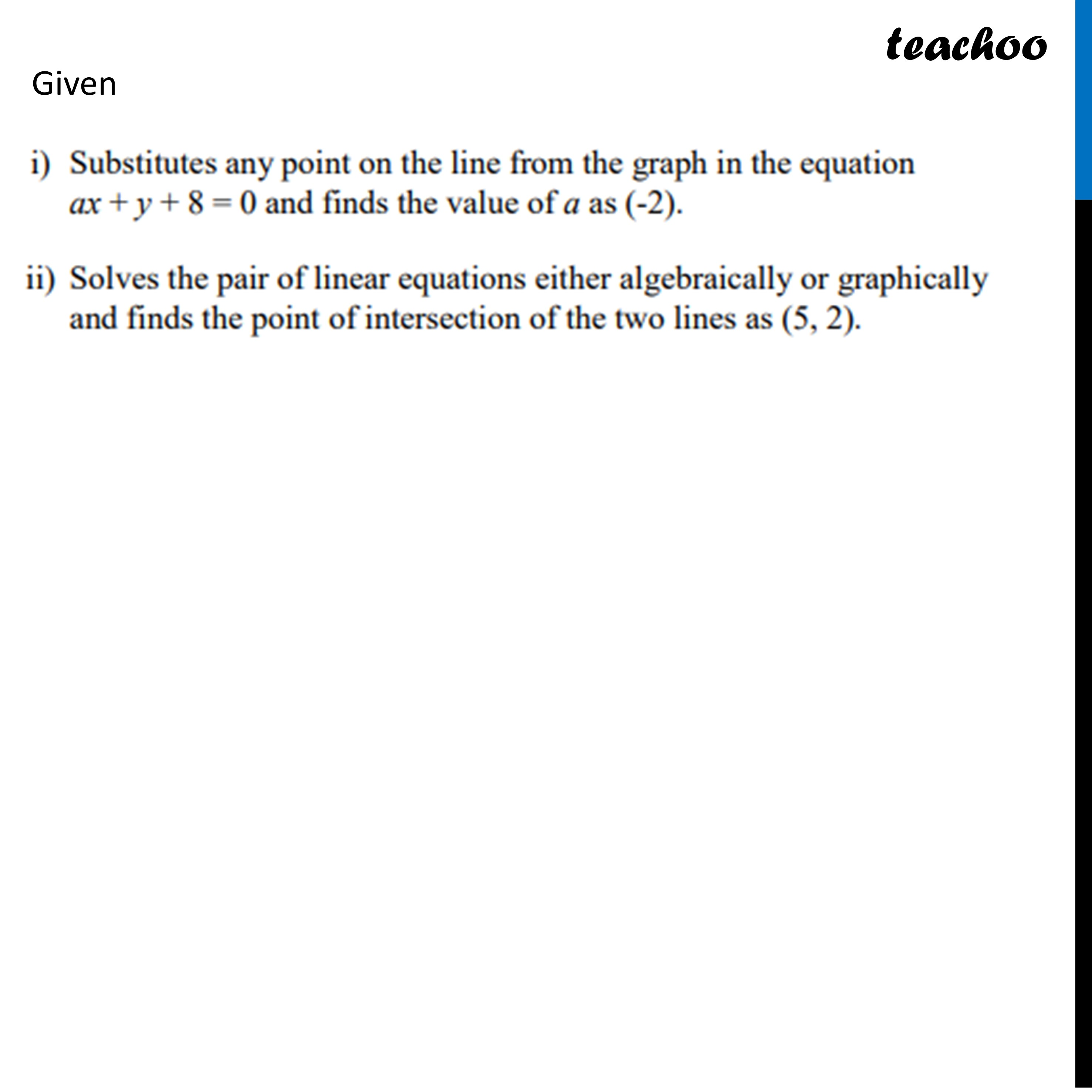Practice Questions CBSE - Maths Class 10 (2023 Boards)

Class 10
Solutions of Sample Papers for Class 10 Boards

## (ii) Find the point of intersection of this line with the line represented by the equation 4x - 3y - 14 = 0.Learn in your speed, with individual attention - Teachoo Maths 1-on-1 Class

### Transcript

The graph of a line represented by the equation ax + y + 8 = 0 is shown in the figure below. (i) Find the value of a. (ii) Find the point of intersection of this line with the line represented by the equation 4x - 3y - 14 = 0. Show your work.